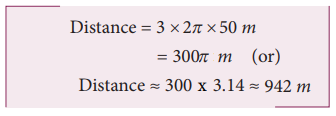Home | | Physics 11th std | Solved Example Problems for Distance and Displacement

# Solved Example Problems for Distance and Displacement

Physics : Kinematics : Solved Example Problems for Distance and Displacement and Vector in Cartesian Coordinate System.

## Solved Example Problems for Distance and Displacement

### Example 2.15

Assume your school is located 2 km away from your home. In the morning you are going to school and in the evening you come back home. In this entire trip what is the distance travelled and the displacement covered?

### SolutionThe displacement covered is zero. It is because your initial and final positions are the same.

But the distance travelled is 4 km.

### Example 2.16

An athlete covers 3 rounds on a circular track of radius 50 m. Calculate the total distance and displacement travelled by him.

SolutionThe total distance the athlete covered =3x circumference of trackThe displacement is zero, since the athlete reaches the same point A after three rounds from where he started.

## Solved Example Problems for Displacement Vector in Cartesian Coordinate System

### Example 2.17

Calculate the displacement vector for a particle moving from a point P to Q as shown below. Calculate the magnitude of displacement.### SolutionTags : Physics , 11th Physics : UNIT 2 : Kinematics
Study Material, Lecturing Notes, Assignment, Reference, Wiki description explanation, brief detail
11th Physics : UNIT 2 : Kinematics : Solved Example Problems for Distance and Displacement | Physics